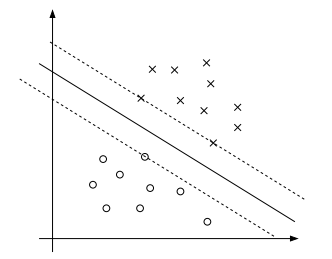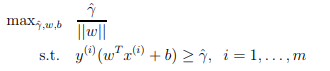## Python Tutorials: Machine Learning in Python

##### Python Tutorials: Get the Machine Learning Skills You Need for Today's Fastest Growing Job
Written by |17-Oct-2020 | 0 Comments | 627 Views

Now we will see how we can implement these algorithms in python. The library that is used in python for the machine learning algorithm is sklearn. This library is very vast and has superiority over all the modules that are available in different languages or frameworks.

To install the sklearn following method is implemented in a command prompt:

 Windows user pip install sklearn Linux or Mac user pip3 install sklearn

So now we are going to apply dummy data in which X  is the data in which height, weight, and shoe size are given, and Y is the label in which 1 and 0 are given one is for male and 0 is for female. Now we are going to make a classifier that will tell either the person is male or female by height, weight, and shoe size as a parameter.

The code is as follows:

 import NumPy as np from sklearn.naive_bayes import GaussianNB   X = np.array([[5.8, 80, 8] , [6.1, 85, 9],[5.7 ,79 ,8],[5.4 ,50 ,6],[5.1 ,55 ,7],[5.3 ,57 ,6]]) y = np.array([1, 1, 1, 0, 0, 0])   model = GaussianNB() model.fit(X,y) print(model.predict([[5.9,90,9]]))

In the first line, we have imported the Numpy, and then from sklearn naive Bayes, we have imported the GaussianNB method. Then we have made fake data and labels fit the data into the model. After the model is trained, we again try to predict the fake data that is not in the data. We have given the data with the intention of males. With the height of 5feet and 9 inches, the weight of 90 kg, and a shoe size of 9 and our model predicted the right male. The output that is generated is one that indicates that it is male.

Similar code can be run with MultinomialNB as follows:

 import NumPy as np from sklearn.naive_bayes import MultinomialNB   X = np.array([[5.8, 80, 8] , [6.1, 85, 9],[5.7 ,79 ,8],[5.4 ,50 ,6],[5.1 ,55 ,7],[5.3 ,57 ,6]]) y = np.array([1, 1, 1, 0, 0, 0])   model = MultinomialNB() model.fit(X,y) print(model.predict([[5.9,90,9]]))

The output is the same because it is a straightforward dataset which can be applied anyhow to any other dataset. But the use of algorithm changes with the type of dataset.

Discriminative Algorithm:

Discriminative models, also called conditional models, are a class of models used in machine learning for modeling the dependence of unobserved (target) variables on observed variables x. Within a probabilistic framework, this is done by modeling the conditional probability distribution p(x|y), which can be used for predicting y from z.

The following are the types of discriminative algorithms:

• Linear Regression
• Logistic Regression
• SVM
• KNN
• Neural networking.

Here we will discuss the only SVM.

Support Vector Machine (SVM):

SVM is the most prominent classifier among individuals. In AI, SVM are supervised learning models with related inclining calculations that investigate information utilized for order and relapse examination. Given a lot of preparing precedents, each set apart as having a place with either of two classifications, an SVM preparing calculation manufactures a model that appoints new guides to one class or the other, making it a non-probabilistic double direct classifier.

An SVM model is a portrayal of the precedents as focuses in space, mapped so the instances of the separate classes are separated by a reasonable distance or gap that is wide as could be expected under the circumstances.

In addition to linear classification, SVM can efficiently perform nonlinear classification by using kernel techniques.

Why SVM is considered to be the best:

SVM is considered the best classifiers among people who are in the field of machine learning due to the maximum margin separating hyperplane. Take an example of linear regression; what linear regression do is to make a straight line between two feature vector x. In contrast to linear regression, what SVM do is to make multiple margin lines and choose the one which has the maximum gap between two feature vector x. Which makes the SVM more reliable and makes the prediction more accurate.

We can show the clear difference graphically as below:Figure 1

As the image depicts that the margin line is far apart from the two feature vectors x

The points with the smallest margins are precisely the ones closest to the decision boundary; here, these are the three points (one negative and two positive examples) that lie on the dashed lines parallel to the decision boundary.

Linear SVM:

We are given a training dataset of n points of the form:

 = (x1, y1), …… ,(xn,yn)

where the x1 till xn are feature vectors, and ith of y indicates the class to which x is a vector.

Any hyperplane can be written as the set of point x satisfying

 = w. x - b = 0

Hard Margin:

On the off chance that the training data information is straightly detachable, we can choose two parallel hyperplanes that separate the two classes of information, with the goal that the separation between them is as vast as could be allowed. The region bounded by these two hyperplanes is called margins.

Mathematically it can be represented as

 = w. x -b = 1 (anything above or on this boundary is of one class) = w. x -b = -1 (anything below or on this boundary is of one class)

Optimal Margin Classifier:

Given a training data set, it appears from our experience that a characteristic purpose is to attempt to discover a choice limit that expands the (geometric) edge since this would mirror a sure arrangement of expectations on the preparation set and a decent "fit" to the training set information.

Precisely, this will result in a classifier that distributes the positive and the negative training examples with a "gap" (geometric margin). For the present, we will accept that we are given a directly detachable preparation set, i.e., that it is conceivable to isolate the positive and negative models utilizing some isolating hyperplane. How we locate the one that accomplishes the most extreme geometric edge? We can represent the accompanying streamlining issue: